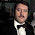## Wednesday, January 15, 2014

### On Euler Equations

Noah Smith calls the Euler Equation "The Equation at the Core of Macro." He explains, "For the uninitiated, the Consumption Euler (pronounced "oiler") Equation is sort of like the Flux Capacitor that powers all modern 'DSGE' macro models." He adds:
"This equation underlies every DSGE model you'll ever see, and drives much of modern macro's idea of how the economy works. So why is Eichenbaum, one of the deans of modern macro, pooh-poohing it?

Simple: Because it doesn't fit the data. The thing is, we can measure people's consumption, and we can measure interest rates. If we make an assumption about people's preferences, we can just go see if the Euler Equation is right or not!"
Noah points to a 2006 paper that uses US data to calculate the interest rate implied by the Euler equation under various assumptions about preferences. The authors compare this Euler equation rate with a money market rate, and find that the two series differ significantly. Noah writes:
"If this paper is right - and one paper is not enough to be conclusive - then essentially all modern DSGE-type macro models currently in use are suspect. The consumption Euler Equation is an important part of nearly any such model, and if it's just wrong, it's hard to see how those models will work... We need a whole literature of papers analyzing whether the Euler Equation is usable or not."
I just want to make the point that Euler equations are not only part of "modern DSGE-type macro models," and that we do have a pretty big empirical literature on the Euler equation. Here are lecture notes from a microeconomics course deriving the Euler equation.

DSGE stands for dynamic stochastic general equilibrium. It is really the D of DSGE that brings in the Euler equations. Optimization problems--which are what a lot of economists and even non-economists solve-- whether deterministic or stochastic, whether partial or general equilibrium, have first-order conditions. Dynamic optimization problems have more than one time period, so you can have first-order conditions in consumption in different time periods. Combine these first-order conditions in consumption with a budget constraint and you get an Euler equation--whether or not you are writing down a DSGE model. The most obvious ways to get rid of the Euler equation are to get rid of optimization (a la Campbell and Mankiw 1991), add other constraints (liquidity constraints, incomplete markets), or pick weird preference relations that make utility non-time-separable (a la Campbell Cochrane 1999).

Euler equations are also foundational in finance and asset pricing. John Cochrane's "Asset Pricing" textbook, used in most graduate-level asset pricing courses, begins with a chapter based on the Euler equation. Here is a chapter by Campbell on Consumption-Based Asset Pricing which makes clear that the Euler equation is at the heart.

A 2012 paper by Sydney Ludvigson, called "Advances in Consumption-Based Asset Pricing:
Empirical Tests," provides a good overview of the empirical literature on consumption-based asset pricing. Ludvigson explains that "early empirical studies found that the model was both formally and informally rejected in a variety of empirical settings." However, "In response to these findings, researchers have altered the standard consumption-based model to account for new preference orderings based on habits or recursive utility, or new restrictions on the dynamics of cash-flow fundamentals, or new market structures based on heterogeneity, incomplete markets, or limited stock market participation." Ludvigson's paper might address some of the issues that commenters to Noah's post brought up.

#### 1 comment:

1.I see Nick Rowe already made essentially this comment on Noah's blog, but perhaps it kind of gets lost in the multiplicity of comments, so I'll say it here. My immediate thought when hearing that empirical studies find the wrong correlation for Euler equations is that there is an identification problem. The studies Noah cites, I presume, make (implicitly or explicitly) the identifying assumption that preferences are constant over time. But if shocks to preferences (and specifically to the time preference parameter) are driving the data, then you would expect interest rates to be higher exactly when people want to consume more. In that case, the Euler equation isn't wrong; it's just not being used properly.

(Of course economists who want to keep clear of the Lucas critique have an incentive to assume constant preferences, because once you admit that preferences can change, you must start to worry that they can be influenced by policy, and probably technology can too, so the prospect for ever having robust structural models starts to diminish.)

Comments appreciated!